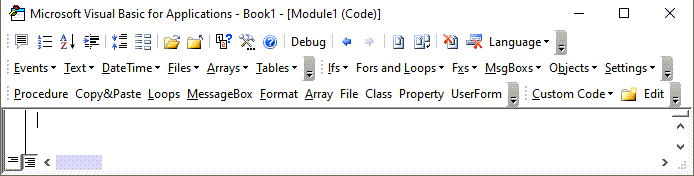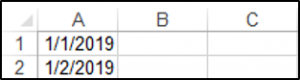# VBA Date Variable

We have already gone over what variables, data types and constants are, in our VBA Data Types – Variables and Constants tutorial. In this tutorial, we are going to cover how to declare a variable as a date.

In VBA, the date data type is stored as a decimal. Both dates and times can be stored in this data type. The VBA date data type can store values ranging from 1 January  100 – 31 December 9999.

## VBA Date Variable

You declare a variable as a date using the Dim keyword:

``Dim dateOne as Date``

Then you can assign a date to the date variable.  There are multiple ways to generate dates to assign to variables:

### Assign Today to Variable

The Date Function will return today’s date:

``````Dim dtToday

dtToday = Date``````

### Assign Now to Variable

The Now Function will return today’s date and current time:

``````Dim dNow

dNow = Now``````

### Assign Time to Variable

The tim will return the current time:

``````Dim tTime

tTime = Time``````

### Assign a Date to Variable with DateSerial

The DateSerial function will return a date based on an input year, month and day:

``````Dim dsDate

dsDate = DateSerial(2010, 11, 11)``````

### Assign a Time to Variable with TimeSerial

The TimeSerial function will return a time, based on an input hour, minute and second:

``````Dim tsTime

tsTime = TimeSerial (10, 10, 45)``````

You must enclose dates with the # or ” when using them in your code, as shown below:

## VBA Coding Made Easy

Stop searching for VBA code online. Learn more about AutoMacro - A VBA Code Builder that allows beginners to code procedures from scratch with minimal coding knowledge and with many time-saving features for all users!### VBA DateValue Function

The VBA DateValue Function can be used to initialize a date. The following code can be used to initialize a date in VBA:

``MsgBox DateValue("1/1/2010")``

### VBA TimeValue Function

The TimeValue Function generates a time:

``MsgBox TimeValue("21:30:57")``

### Excel VBA Date Variable Example

This procedure demonstrates how to create date variables, assign them values, and output them into an Excel worksheet:

``````Sub DeclaringAVariableAsADate()

Dim dateOne As Date
Dim dateTwo As Date

dateOne = #1/1/2019#
dateTwo = "1/2/2019"

Range("A1").Value = dateOne
Range("A2").Value = dateTwo

End Sub``````

The result is:You can learn more about Date Functions in this tutorial.

VBA Programming | Code Generator does work for you!

## VBA Date Variable in Access

The Date Variable works exactly the same in Access VBA as in Excel VBA.

This procedure demonstrates how to create a date variable and update a field in an access table with the value.

``````Sub DeclaringAVariableAsADate()
Dim dtWork As Date
dtWork = #05/10/2020#
DoCmd.RunSql "UPDATE tblJobs SET WorkDate = #" & dtWork & "# WHERE JobNo = 6"
End Sub``````